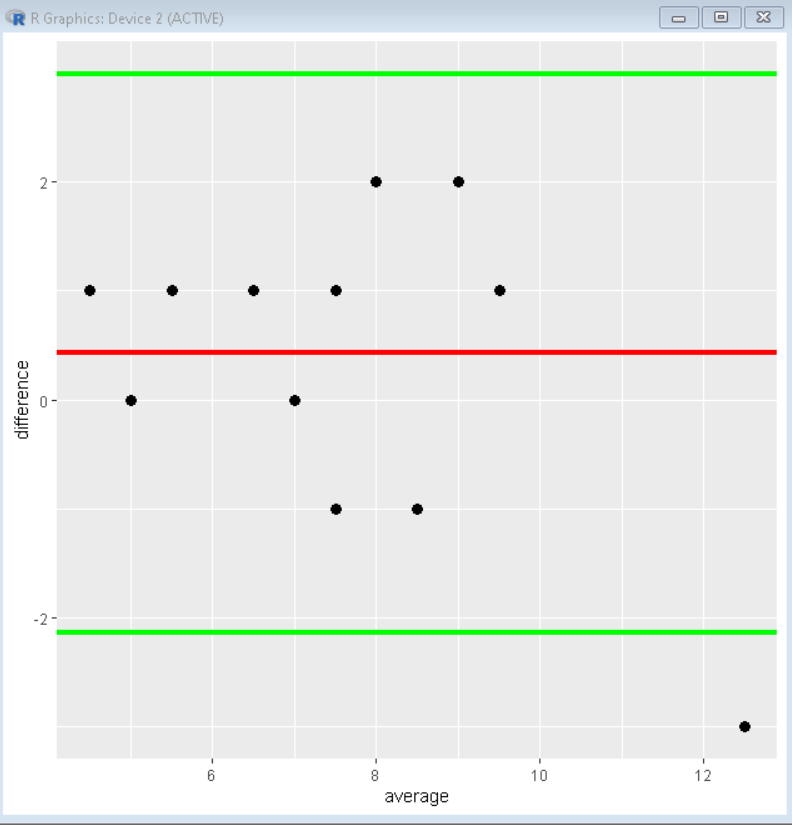# How to Create a Bland-Altman Plot in R?

• Last Updated : 04 Jan, 2022

In this article, we will discuss how to create a Bland-Altman Plot in the R programming Language.

The Bland-Altman plot helps us to visualize the difference in measurements between two different measurement techniques. It is used vastly in the field of biochemistry. It is useful for determining how similar two instruments/techniques are at measuring the same construct in chemical reactions.

## Stepwise Implementation

Step 1: Create sample dataframe

To create a sample data frame in the R language, we can either import dataset from a CSV file by using the read.csv() function or can create our own data frame by using the data.frame() function.

Step 2: Calculate the difference in measurements

Next, we will add two new columns to data frame that will store the average and difference of both variables for plotting afterward. We will use the assignment function for that purpose.

Syntax:

Sample_Data\$average <- rowMeans( Sample_Data )

Sample_Data\$difference <- Sample_Data\$var1 – Sample_Data\$var2

Step 3: Calculate mean difference and Limits of the confidence interval

To calculate the mean difference, we will use the mean() function of the R Language. To calculate the lower and upper limit of 90% confidence interval, we will use the sd() function.

Syntax:

mean_diffe

e <- mean( Sample_Data\$difference )

lower_limit <- mean_difference – 1.91*sd( Sample_Data\$difference )

upper_limit <- mean_difference + 1.91*sd( Sample_Data\$difference )

Step 4: Plot the mean difference, Confidence interval, and scatter plot

To plot the Bland-Altman Plot we will use the ggplot() function of the ggplot2 package library along with the geom_hline() function for mean_difference and confidence interval.

Syntax:

ggplot( Sample_Data, aes( x = average, y = difference ) ) + geom_point( ) +

geom_hline( yintercept = mean_differnce ) + geom_hline( yintercept = lower_limit ) +

geom_hline( yintercept = upper_limit )

This gives the Bland-Altmon Plot finally.

Example: A sample Bland-Altmon Plot

## R

 `# create sample data``Sample_Data <- ``data.frame``(``  ``var1=``c``(5, 5, 5, 6, 6, 7, 7, 7, 8, 8, 9, 10, 10, 11),``  ``var2=``c``(4, 4, 5, 5, 5, 7, 8, 6, 9, 7, 7, 8, 9, 14))`` ` `# create new column for average measurement``Sample_Data\$average <- ``rowMeans``(Sample_Data) `` ` `# create new column for difference measurement``Sample_Data\$difference <- Sample_Data\$var1 - Sample_Data\$var2`` ` `# calculate mean difference``mean_difference <- ``mean``(Sample_Data\$difference)`` ` `# calculate uppr and lower limits of the ``# Confidence interval of 90%``lower_limit <- mean_difference - 1.91*``sd``( Sample_Data\$difference )``upper_limit <- mean_difference + 1.91*``sd``( Sample_Data\$difference )`` ` `# load library ggplot2``library``(ggplot2)`` ` `# Plot the Bland-Altmon Plot``ggplot``(Sample_Data, ``aes``(x = average, y = difference)) +``  ``geom_point``(size=3) +``  ``geom_hline``(yintercept = mean_difference, color= ``"red"``, lwd=1.5) +``  ``geom_hline``(yintercept = lower_limit, color = ``"green"``, lwd=1.5) +``  ``geom_hline``(yintercept = upper_limit, color = ``"green"``, lwd=1.5)`

Output:My Personal Notes arrow_drop_up